# T.DIST Function

Calculates the student's t-distribution

## T Distribution and T Value Excel Function

The T.DIST Function is categorized under Excel Statistical functions. It will calculate T distribution. The T distribution is a continuous probability distribution that is frequently used in testing hypotheses on small sample data sets. This guide will show you how to use the T Distribution Excel formula and T Value Excel function step by step.

As a financial analyst, T.DIST is used in portfolio risk analysis. It is used to model asset returns to take care of heavy tails. This is so as the T distribution includes fatter tails, which gives higher values of value-at-risk at high confidence levels.

The T.DIST function was introduced in Excel 2010, hence, is not available in earlier versions.

### The Formula

=T.DIST(x,deg_freedom,tails)

The T.DIST function uses the following arguments:

1. X (required argument) – This is the numeric value at which we wish to evaluate the T Distribution.
2. Deg_freedom (required argument) – An integer that indicates the number of degrees of freedom.
3. Tails (required argument) – This specifies the number of distribution tails that would be returned. If tails =1, T.DIST returns the one-tailed distribution. If tails = 2, T.DIST returns the two-tailed distribution.

### How to use the T Distribution Excel function

To understand the uses of the T.DIST function, let us consider a few examples:

#### Example 1

Suppose we are given the following data: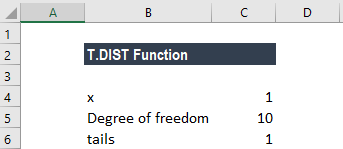If we want to calculate the value of the function at x = -1, it can be done using the formula below: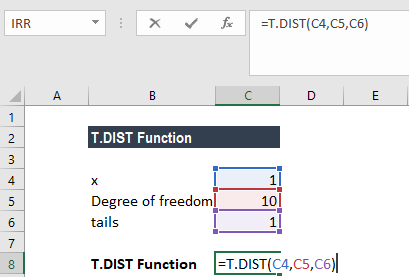We get the result below: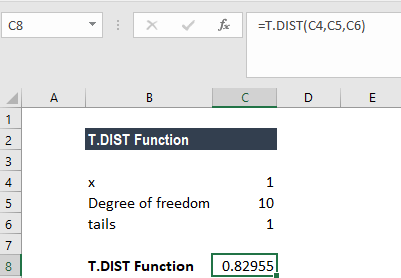The result we get is 82.95%.

If we want to calculate the value of the function at x = 1, it can be done using the formula below: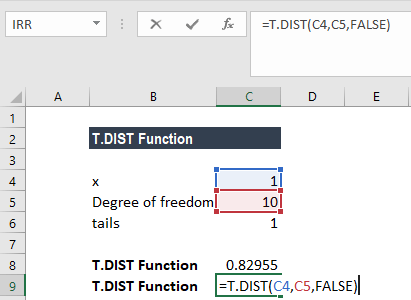We get the result below: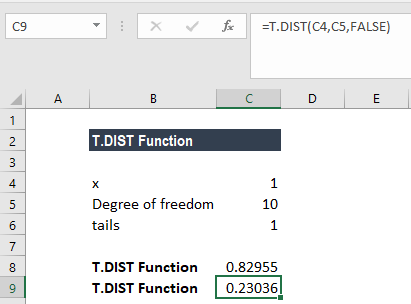The result we get is 23.036%.

### Things to remember about the T Value Excel function

1. #NUM! errorOccurs when:
1. The given cumulative is TRUE and deg_freedom < 1.
2. The given cumulative is FALSE and deg_freedom < 0.
2. VALUE! errorOccurs when the given arguments are non-numeric.
3. The deg_freedom and tails arguments are truncated to integers.
4. #DIV/0 errorOccurs when the given cumulative is FALSE and deg_freedom is equal to 0.

Thanks for reading CFI’s guide to the T Distribution Excel function and the T Value Excel function! By taking the time to learn and master these functions, you’ll significantly speed up your financial analysis. To learn more, check out these additional CFI resources:

• Excel Functions for Finance